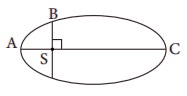Home | Multiple Choice Questions - Physics: Gravitation

# Multiple Choice Questions - Physics: Gravitation

1 mark answers, Multiple Choice Questions - Physics: Gravitation

Gravitation (Physics)

Multiple Choice Questions

1.   The linear momentum and position vector of the planet is perpendicular to each other at

(a) perihelion and aphelion

(b) at all points

(c) only at perihelion

(d) no point

Answer : a) perihelion and aphelion

Solution :

L = mv ├Ś r

Here, L, r and v are mutually perpendicular. So. A and P ate 90┬░ in two position because it is elliptical orbit.2.   If the masses of the Earth and Sun suddenly double, the gravitational force between them will

(a) remain the same

(b) increase 2 times

(c) increase 4 times

(d) decrease 2 times

Answer : c) increase 4 times

Solution :

If mass of earth and sun is doubled then the force?

F1 = GMEMS / R2

F2 = [ G(2ME)(2MS) ] / [ R] = 4GMEMS / R2

F2 = 4F1

Answer : a) r2 / r1

Solution :

L1 = mv1 , r1 & L2 = mv2 r2

L= L2

mv1 r1 = mv2 r2

V1 / V2 = r2 / r1 (Ōł┤ v ŌłØ 1 / r)

3.   A planet moving along an elliptical orbit is closest to the Sun at distance r1 and farthest away at a distance of r2. If v1 and v2 are linear speeds at these points respectively. Then the ratio V1/V2 isAnswer : a) r2 / r1

Solution :

L1 = mv1 , r1 & L2 = mv2 r2

L= L2

mv1 r1 = mv2 r2

V1 / V2 = r2 / r1 (Ōł┤ v ŌłØ 1 / r)

4. The time period of a satellite orbiting Earth in a cirular orbit is independent of .

(a) Radius of the orbit

(b) The mass of the satellite

(c) Both the mass and radius of the orbit

(d) Neither the mass nor the radius of its orbit

Answer : b) The mass of the satellite

Solution :

T = 2ŽĆ ŌłÜ[R╔ø / g]

Independent of mass of the satellite.

5. If the distance between the Earth and Sun were to be doubled from its present value, the number of days in a year would be

(a)  64.5

(b) 1032

(c)  182.5

(d)  730

Answer : b) 1032

Solution :

b) T2 ŌłØ a3

T1= const. a13 ŌćÆ T1/ a1= const

T2= const. a23 ŌćÆ T2/ a2= const

(1) = (2)

T1/ a13 = T2/ a23

a1 = a

a2 = 2a

T22 = T1(2a / a )3

T22 = 2 ├Ś 2 ├Ś 2 ├Ś T12

T2 = ŌłÜ[4 ├Ś 2] ├Ś 365

T2 = 2 ├Ś ŌłÜ2 ├Ś 365

T2 = 1032.22 days

6. According to KeplerŌĆÖs second law, the radial vector to a planet from the Sun sweeps out equal areas in equal intervals of time. This law is a consequence of

(a) conservation of linear momentum

(b) conservation of angular momentum

(c) conservation of energy

(d) conservation of kinetic energy

Answer : b) Conservation of angular momentum

7. The gravitational potential energy of the Moon with respect to Earth is

(a) always positive

(b) always negative

(c) can be positive or negative

(d) always zero

Answer : b) always negative

8. The kinetic energies of a planet in an elliptical orbit about the Sun, at positions A, B and C are KA, KB and KC respectively. AC is the major axis and SB is perpendicular to AC at the position of the Sun S as shown in the figure. Then(a) KA > KB >KC

(b) KB < KA <KC

(c) KA < KB <KC

(d) KB > KA >KC

Solution :

KE = GmEmS / (RE + h)

KE ŌłØ 1 / ReŌåō

KA > KB > KC

9. The work done by the SunŌĆÖs gravitational force on the Earth is

(a)  always zero

(b)  always positive

(c)  can be positive or negative

(d)  always negative

Answer : c) can be positive or negative

10. If the mass and radius of the Earth are both doubled, then the acceleration due to gravity g'

(a) remains same

(b) g/2

(c)  2g

(d)  4g

Answer: b) g / 2

Solution:

g = GM / R2

g = G(2M) / (2R)2 = 2GM / 4R2 = 1/2 (GM / R2)

g = g / 2

11. The magnitude of the SunŌĆÖs gravitational field as experienced by Earth is

(a)  same over the year

(b)  decreases in the month of January and increases in the month of July

(c)  decreases in the month of July and increases in the month of January

(d)  increases during day time and decreases during night time.

Answer : c) decreases in the month of July and increases in the month of January

12. If a person moves from Chennai to Trichy, his weight

(a)  increases

(b)  decreases

(c)  remains same

(d)  increases and then decreases

Ans : b) decreases

Solution:Chennai 13o

Trichy 11o

ŌłØ 1 / R

13. An object of mass 10 kg is hanging on a spring scale which is attached to the roof of a lift. If the lift is in free fall, the reading in the spring scale is

(a)  98 N

(b)  zero

(c)  49 N

(d)  9.8 N

14. If the acceleration due to gravity becomes 4 times its original value, then escape speed

(a)  remains same

(b)  2 times of original value

(c)  becomes halved

(d)  4 times of original value

Answer : b) 2 times of original value

Solution :

Ve = ŌłÜ[2gR]

g = 9.8ms-2 .

V┬┤e = ŌłÜ[2(4g)R]

V┬┤e = 2ŌłÜ(2gR) = 2Ve

15. The kinetic energy of the satellite orbiting around the Earth is

(a)  equal to potential energy

(b)  less than potential energy

(c)  greater than kinetic energy

(e)  zero

Answer : b) Less than potential energy

Solution :

KE = 1/2 [ GmEmS / (Re + h) ]

U = ŌłÆ GmEmS / (RE + h)

Ōł┤ KE = U / 2

KE < U

1) a    2) c    3) a    4) b   5) b

6) b   7) b   8) a    9) c    10) b

11) c  12) a  13) b 14) b 15) b

Tags : with answer , 11th Physics : UNIT 6 : Gravitation
Study Material, Lecturing Notes, Assignment, Reference, Wiki description explanation, brief detail
11th Physics : UNIT 6 : Gravitation : Multiple Choice Questions - Physics: Gravitation | with answer